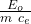## Four materials have the same initial temperature and mass, if all the four materials absorbed 5000 Joules of heat which material has the hig

Question

Four materials have the same initial temperature and mass, if all the four materials absorbed 5000 Joules of heat which material has the highest temperature?
Glass

Copper

Gold

in progress 0
2 months 2021-07-24T01:25:23+00:00 1 Answers 3 views 0

reaches a higher temperature is LEAD.

Explanation:

In this exercise we have a calorimeter problem, in which the initial energy is absorbed by each body

E₀ = m c_e ΔT

where E₀ = 5000 J

ΔT =the specific heats for each material are tabulated

material   c_e (J / kg ºC)

glass           837

copper       387

gold            129

As they indicate that the mass of the materials is the same, the one with the greatest temperature difference is the one with the least specific heat, consequently the one that reaches a higher temperature is LEAD.

let’s calculate for a mass of 1 kg

glass

ΔT = 5000/(1 837)

ΔT = 6ºC